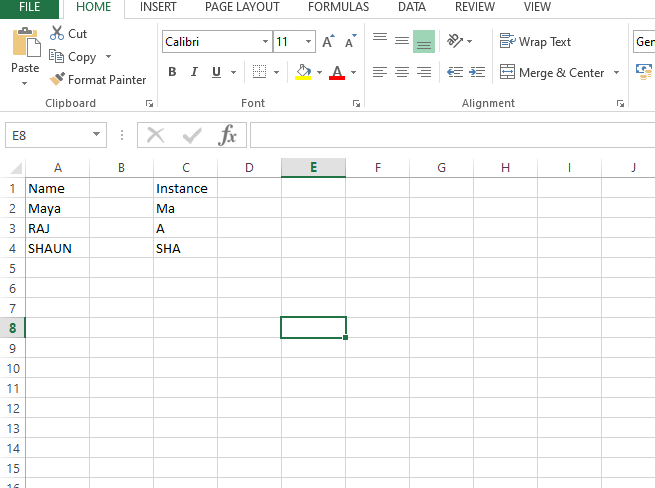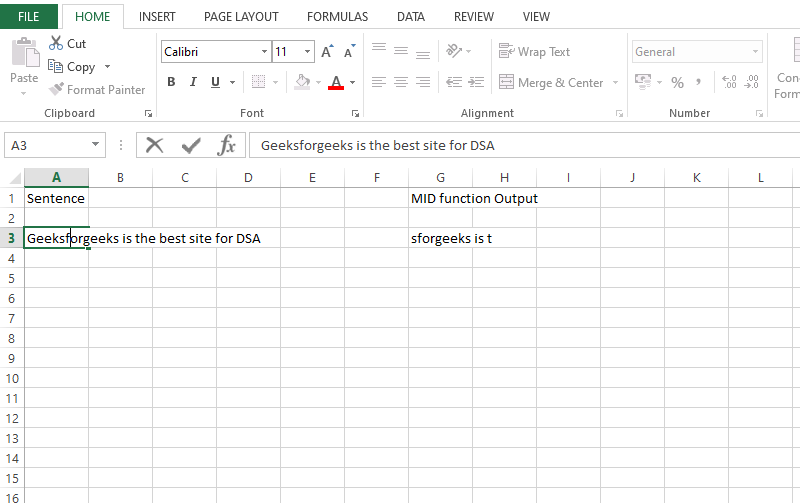# MID Function in Excel

• Last Updated : 06 Sep, 2021

If you use programming languages, like python, you must have used a function called string slicing, which extracts a part of the string and can directly print it, or store a result. The same functionality can be achieved in Excel when we use Excel’s MID function.

The MID function can return a part of the string provided to it. Let us see the working of this function.

### Mind Function in Excel:

This function can be used to slice a given string and returning only a part of that string. It considers a starting point, which will be the starting index of the string, from which we want to print. It will consist of the string that we want to slice. It can be a string provided in double quotes(” “), or it can be a reference to a cell on which slicing is to be done. It will also consist of the length of the characters, which will indicate how many characters we want to print taking reference from the starting index.

Syntax:

`=MID(Text to be sliced, starting index, length of the string)`

Now we will see a few examples of MID function.

Example:Here,we used MID function 3 times on cells,i.e on MAYA,RAJ,SHAUN.Now, we will see individual 3 formulas for these three:

1. In cell A2(MAYA), we applied–> MID(A2,1,2): Here, we used A2 as our text to be sliced. We will start from the 1st index, ie the very first element, which is “M”.The length of the string is kept as 2, so the string will be sliced till the 2nd index of the string, which is “A”. This will result in the output “MA”.
2. In cell A3(RAJ), we applied–> MID(A3,2,1): Here, we used A3 as our text to be sliced. We will start from the 2nd index, which is “A”.The length of the string is kept as 1, so the sliced string will be till this index only. This will result in the output “A”.
3.  In cell A4(SHAUN), we applied–> MID(A4,1,3):  Here, we used A4 as our text to be sliced. We will start from 1st index, ie the very first element, which is “S”.The length of the string is kept as 3, so the string will be sliced till the 3rd index of the string, which is “A”.  This will result in the output “SHA”.

It is not necessary to provide just a word to be sliced, we can also use a sentence, that can be sliced by using the MID function. The only thing to keep in mind is that the spaces between the words in a sentence will also be counted as an index.

Example 2:Here we applied the MID function to the A3 cell, which is a sentence, and we obtained a sliced string, which is between the A3 and the B3 cell. Now, let us see how the MID function was applied.

`=MID(A3,5,14)`

Here, the MID function will slice the string stored in A3 column. The starting point of slicing is from the 5th index, which is “s”.Now, as previously discussed, the spaces between the words in a sentence, also count as an index, so the length of 14 also included the space between “s” and “t”. Therefore the outcome is “sforgeeks is t”.

Attention reader! Don’t stop learning now. If you are an Excel beginner (or an intermediate) and want to learn Excel, Geeksforgeeks brings the perfect course for you to start, Diving Into Excel

My Personal Notes arrow_drop_up